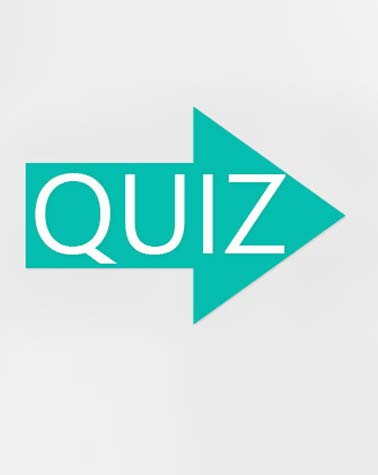# Grade 8 Chapter 6 Physical Science

20 Questions | Attempts: 101SettingsChapter Six deals with Chemical Reactions

• 1.
Chemistry is...
• A.

A characteristic of a substance that can be observed without changing the substance into another substance.

• B.

The study of matter and how matter changes.

• C.

Anything that has mass and takes up space.

• D.

A rapid reaction between oxygen and a substance called a fuel.

• 2.
What type of reaction is FeS + 2HCl à Fe Cl2 + H2S?
• A.

A synthesis reaction.

• B.

A decomposition reaction

• C.

A single displacement reaction

• D.

A double displacement reaction

• 3.
Which of the following is NOT a correct inference that you can make about the  burning of gasoline?
• A.

Chemical bonds are broken and others are formed.

• B.

The reaction is a physical change.

• C.

New substances are produced.

• D.

Energy is released.

• 4.
A chemical equation that shows the same number of each kind of atom on both sides of the equation is said to be...
• A.

Balanced

• B.

Unbalanced

• C.

An incomplete reaction

• D.

A replacement reaction

• 5.
Anything that has mass and takes up space is considered...
• A.

Matter

• B.

Energy

• C.

Chemistry

• D.

Endothermic

• 6.
What happens when chemical bonds break and new bonds form?
• A.

A physical change.

• B.

A chemical change.

• C.

Matter is destroyed.

• D.

Surface area increases

• 7.
CaCO3   represents a chemical ...
• A.

Symbol.

• B.

Formula.

• C.

Subscript.

• D.

Reaction.

• 8.
The only sure evidence for a chemical reaction is...
• A.

The formation of a gas.

• B.

A color change.

• C.

The production of one or more new substances.

• D.

Changes in properties.

• 9.
A shorter, easier way to show chemical reactions, using symbols instead of words, is called a...
• A.

Chemical equation

• B.

Chemical formula

• C.

Symbol

• D.

Subscript

• 10.
The substances listed on the left side of a chemical equation are the...
• A.

Products

• B.

Coefficients.

• C.

Precipitates

• D.

Reactants

• 11.
In chemical reactions, what does the principle of conservation of matter mean?
• A.

Matter is not created or destroyed.

• B.

The total mass of the reactants is greater than the total mass of the products.

• C.

The total mass of the reactants is less than the total mass of the products.

• D.

Matter is not changed.

• 12.
Which of the following is a balanced equation?
• A.

H2O ---> H2O + O2

• B.

2Fe2O3 + 3C --> 4Fe + 3CO2

• C.

SO2 + O2 + 2H2O --> 4H2SO4

• D.

2Mg + HCl --> MgCl2 + H2

• 13.
Which two processes could be considered opposites?
• A.

A decomposition reaction and a single replacement reaction.

• B.

A single replacement reaction and a double replacement reaction.

• C.

A precipitate reaction and a synthesis reaction

• D.

A synthesis reaction and a decomposition reaction

• 14.
A bottle of hydrogen peroxide that eventually turns into a bottle of water and oxygen gas is an example of a ....
• A.

Synthesis reaction

• B.

Decomposition reaction

• C.

Replacement reaction

• D.

Precipitate reaction

• 15.
A chemical reaction that absorbs energy in the form of heat is described as...
• A.

Endothermic

• B.

Exothermic

• C.

Combustion

• D.

Unbalanced

• 16.
Which is the correct chemical equation for the following statement? Sodium reacts with oxygen in a 2 to 1 ratio to produce sodium oxide?
• A.

2Na + O2 --- Na2O

• B.

NOT THIS ONE

• C.

NOT THIS ONE

• D.

NOT THIS ONE

• 17.
When a chemical reaction takes place in an open system....
• A.

Matter can enter from or escape to the surroundings.

• B.

Matter is not allowed to enter from or escape to the surroundings.

• C.

Matter can enter from the surroundings, but can not escape to the surroundings.

• D.

Matter can not move at all.

• 18.
In a chemical equation, numbers often appear in front of a chemical formula. These numbers tell you the...
• A.

Number of atoms in each molecule in the reaction.

• B.

Number of elements in the reaction.

• C.

Number of molecules or atoms of each substance in the reaction.

• D.

Number of molecules in each atom in the reaction.

• 19.
Chemicals that act as biological catalysts by speeding up reactions in living things are...
• A.

Inhibitors

• B.

Enzymes

• C.

Fuels

• D.

Reactants

• 20.
The number placed below an element's symbol in a chemical formula is called a....
• A.

Subscript

• B.

Coefficient

• C.

Reactant

• D.

Product

## Related TopicsBack to top
×

Wait!
Here's an interesting quiz for you.